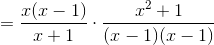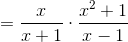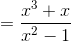## Example Questions

### Example Question #1 : How To Multiply Rational Expressions

Multiply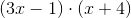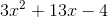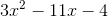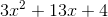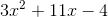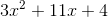Explanation:

When multiplying two binomials, it's best to remember the mnemonic FOIL, which stands for First, Outer, Inner, and Last. Going in the order of FOIL, we multiply the first term of each binomial, then the outer terms of the expression, then the inner terms of the expression, and finally the last term of each binomial. For this question we get: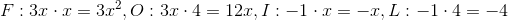Now that we have 'FOILed' our expression, we simply add our results to get the final answer.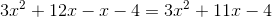### Example Question #1 : How To Multiply Rational Expressions

Give the product in simplified form: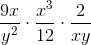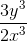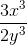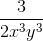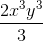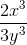Explanation:

Use cross-cancellation as follows: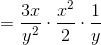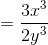### Example Question #2 : How To Multiply Rational Expressions

Give the product in simplified form: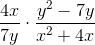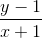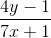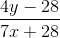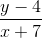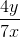Explanation:

Factor the numerator and denominator in the second fraction, cross-cancel common factors, then multiply out.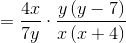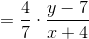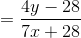### Example Question #3 : How To Multiply Rational Expressions

Give the product in simplified form: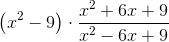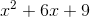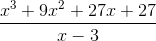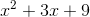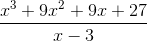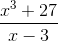Explanation:

Factor the first polynomial and the numerator and denominator in the second fraction, cross-cancel common factors, then multiply out.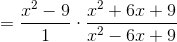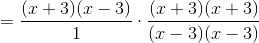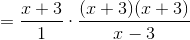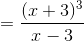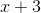can be cubed using the cube of a binomial pattern: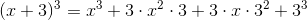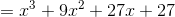so the above rational expression can be rewritten as### Example Question #4 : How To Multiply Rational Expressions

Give the product in simplified form: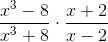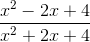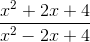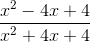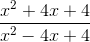Explanation:

Factor the numerator and denominator in the first fraction, cross-cancel common factors, then multiply out.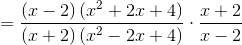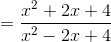### Example Question #5 : How To Multiply Rational Expressions

Give the product in simplified form: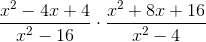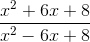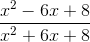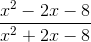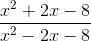Explanation:

Factor the numerators and denominators, cross-cancel common factors, then multiply out. Both numerators are perfect square trinomials; both denominators are differences of squares.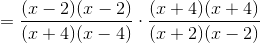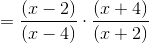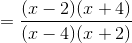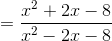### Example Question #6 : How To Multiply Rational Expressions

Give the product in simplified form: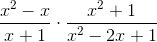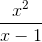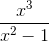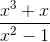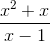Explanation:

Factor the numerators and denominators, cross-cancel common factors, then multiply out. Note that the second numerator is prime.